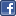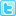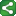Microsoft Excel Resource Center Free Macros, Formulas, Functions, Tutorials, Downloads, Add-Ins & More!
 Monte Carlo Tutorial power4XL Home
 Contents

Modeling normal distributions with the Excel Monte Carlo worksheet-only approachShareThis

Now we'll move onto an essential building block of Monte Carlo simulations: how to model normal distribution curves.  Since we didn't cover this in the earlier "worksheet based simulations" section of this tutorial, we'll cover that here, in addition to two VBA approaches to the same problem.  This set of examples is intended to tie together and reinforce many of the concepts we've covered so far throughout the entire tutorial, in addition to introducing the normal distribution modeling technique.

At the heart of the technique is the worksheet function NormInv().  The function takes the three arguments probability, mean, standard deviation.  So, to generate random numbers with a mean of 10 and standard deviation of 3 is as simple as entering the following formula into a worksheet cell:

=NormInv(Rand(), 10, 3)

In the three examples that follow, we'll use the Scholastic Aptitude Test (SAT) as the basis of our problem.  We want to model a set of SAT scores.

Currently the three-section SAT is calibrated to have a mean total score of 1500 with a standard deviation of 300.  The minimum score is 600 and the maximum is 2400.  (Each section has a minimum of 200 and maximum of 800, mean of 500 and standard deviation of 100).  Every SAT score is a multiple of 10 (i.e., 1240, 1250, etc.)

Thus the input screen for our worksheet-only SAT model is quite straightforward:

Calculating the SAT score using the NormInv() function requires an addition step, since we need to set the minimum and maximum scores to 600 and 2400, respectively, and make sure each score is a multiple of 10.  The following line of code gives a normally-distributed SAT score according to these requirements:

=MIN(MAX(ROUND(NORMINV(RAND(),SAT1Mean,SAT1StdDev)/10,0)*10,600),2400)

where "SAT1Mean" and "SAT1StdDev" are named ranges referring to cells D14 and D15, respectively.

So, using the "worksheet-only" Excel Monte Carlo method, we'll enter that formula into a series of cells, each cell generating one datapoint (or iteration) of the model.  Since our desired final results will be displayed in a histogram, grouping scores rounded to the nearest multiple of 100, we'll add a score "Range" column next to the raw score containing the formula:

=ROUND(D20/100,0)*100

with the "D20" reference changing accordingly for each iteration of the model.An alternative to the separate "Score" and "Range" fields would have been to combine the two formulas into a single one, performing the entire calculation in a single cell.  The formula would read:

=MIN(MAX(ROUND(NORMINV(RAND(),SAT1Mean,SAT1StdDev)/100,0)*100,600),2400)

A minor difference is the first versus second approach is that the first approach rounds scores ending in "50" to the next higher 100, while the second approach rounds down.

Prev | Contents | Next

Copyright notice:  This site and all content, including computer code and spreadsheet examples, are copyright 2006 by Fritz Dooley.  License is granted for individual users to download examples and to copy code directly into user's spreadsheets and Visual Basic for Applications files.  Users may not redistribute code in any way.  Providing hyperlinks to this web site is encouraged, but posting code and examples on other web sites is expressly forbidden.  "Microsoft" is a registered trademark of Microsoft Corporation.   Neither this web site nor Fritz Dooley is affiliated with Microsoft Corporation.